MORE IN Applied Mathematics 2
MU First Year Engineering (Semester 2)
Applied Mathematics 2
December 2012
Total marks: --
Total time: --
INSTRUCTIONS
(1) Assume appropriate data and state your reasons
(2) Marks are given to the right of every question
(3) Draw neat diagrams wherever necessary

1(a) Using Taylor's series, find y(0.4) where dy/dx = 1 + xy with y(0) = 2.
3 M
1(b) Find the complementary function of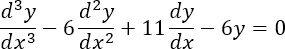3 M
1(c) Evaluate the following: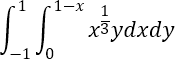3 M
1(d) Evaluate the following: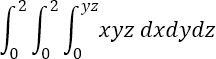3 M
1(e) Show that the following holds true: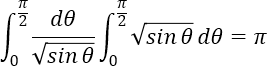4 M
1(f) Using Euler's method, solve: dy/dx = x + y, y(0) =1. Find the value of y at x=1, taking h = 0.2
4 M

2(a) Evaluate the following: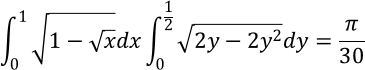6 M
2(b) Use Runge-Kutta method of fourth order to solve dy/dx=1/(x+y); y(0)=1. Find y(0.2) with h=0.1
6 M
2(c) Solve the following: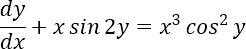8 M

3(a) Solve the following: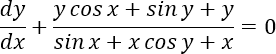6 M
3(b) Solve the following using variation of parameters.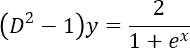6 M
3(c) Evaluate the following: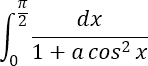and hence deduce that: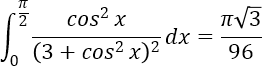8 M

4(a) Solve the following: (xy3+y)dx+2(x2 y2+x+y4 )dy=0
6 M
4(b) Solve the following: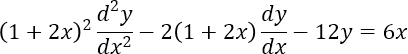6 M
4(c) Solve the following: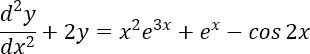8 M

5(a) In a circuit containing Inductance L, Resistance R, and Voltage E, the current i is L di/dt+Ri=E. Find i at time t. At t=0,i=0, L,R,E are constants.
6 M
5(b) Change the order of integration: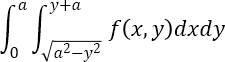6 M
5(c) Evaluate the following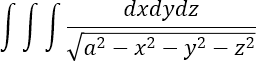over the volume of a sphere x2 + y2 + z2 = a2
8 M

6(a) Find the length of the parabola x2 = 4y which lies inside the circle x2 + y2 = 6y.
6 M
6(b) Change into polar and evaluate: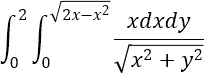6 M
6(c) Evaluate the following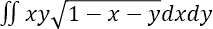over the area of the triangle formed by x = 0, y = 0, x + y = 1.
8 M

7(a) Change the order of integration and evaluate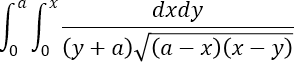6 M
7(b) Find the area outside the circle r=a and inside the cardioid r=a(1+cosθ)
6 M
7(c) Find the volume common to the cylinders: x2 + y2 = a2, x2 + z2 = a2
8 M

More question papers from Applied Mathematics 2